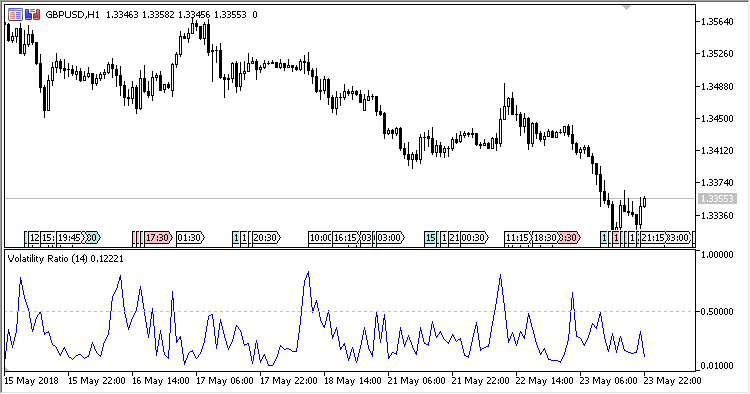# Volatility_Ratio – indicator for MetaTrader 5

Indicator Volatility_Ratio is an attempt to find trading ranges and trend return points.

It works within the range of 0.01-1.0 only. At the values above 0.5, it indicates the probability of the current trend reversal.

It has one input parameter:

• Period – calculation period.
Related Posts

Calculations:

`VR[i] = tr[i] / pr[i]`

where:

`tr[i] = Max(hl[i], hc[i], lc[i])`
```hl[i] = High[i] - Low[i]
hc[i] = Abs(High[i] - Close[i-1])
lc[i] = Abs(Low[i] - Close[i-1])
```
`pr[i] = Max(MH, Open[i-Period+1]) - Min(ML, Open[i-Period+1])`
`MH and ML are the highest and the lowest price over the Period, respectively.`